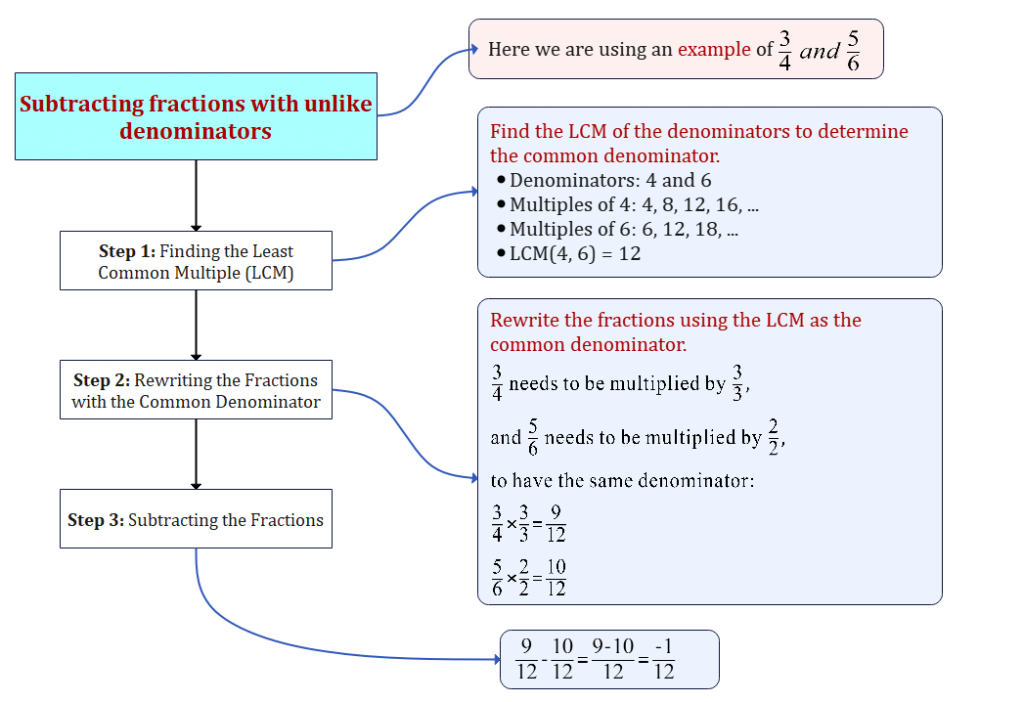Home » Maths » Subtracting fractions with unlike denominators

# Subtracting fractions with unlike denominators

## Subtracting fractions with unlike denominators

### Definitions and Concepts

#### Fraction

• A fraction represents a part of a whole and consists of a numerator and a denominator. For example, $\frac{a}{b}$, where $a$ is the numerator and $b$ is the denominator.

#### Unlike Denominators

• Two fractions have unlike denominators if the denominators are not the same.

### Subtracting Fractions with Unlike Denominators

#### Step 1: Finding the Least Common Multiple (LCM)

To subtract fractions with unlike denominators, find the least common multiple of the denominators.

##### Example:

LCM of 4 and 6:

$\text{LCM}(4, 6) = 12$

#### Step 2: Rewriting the Fractions with the Common Denominator

Next, rewrite both fractions using the LCM as the common denominator.

##### Example:

Rewrite $\frac{3}{4}$ and $\frac{5}{6}$ with 12 as the common denominator.

$\frac{3}{4} = \frac{3 \times 3}{4 \times 3} = \frac{9}{12}$
$\frac{5}{6} = \frac{5 \times 2}{6 \times 2} = \frac{10}{12}$

#### Step 3: Subtracting the Fractions

Subtract the numerators and place the result over the common denominator.

$\frac{9}{12} – \frac{10}{12} = \frac{9 – 10}{12} = \frac{-1}{12}$## Solved Examples

let us have a look at two examples of subtracting fractions with unlike denominators.

### Example 1: Subtracting $\frac{2}{7}$ from $\frac{3}{5}$

#### Step 1: Finding the Least Common Multiple (LCM) Find the LCM of 7 and 5:

$\text{LCM}(7, 5) = 35$

#### Step 2: Rewriting the Fractions with the Common Denominator

$\frac{2}{7} = \frac{2 \times 5}{7 \times 5} = \frac{10}{35}$
$\frac{3}{5} = \frac{3 \times 7}{5 \times 7} = \frac{21}{35}$

#### Step 3: Subtracting the Fractions

$\frac{3}{5} – \frac{2}{7} = \frac{21}{35} – \frac{10}{35} = \frac{11}{35}$

### Example 2: Subtracting $\frac{7}{8}$ from $\frac{3}{5}$

#### Step 1: Finding the Least Common Multiple (LCM)

$\text{LCM}(8, 5) = 40$

#### Step 2: Rewriting the Fractions with the Common Denominator

$\frac{7}{8} = \frac{35}{40}$
$\frac{3}{5} = \frac{24}{40}$

#### Step 3: Subtracting the Fractions

$\frac{3}{5} – \frac{7}{8} = \frac{-11}{40}$

These two examples shows the methodical approach of subtracting fractions with unlike denominators. By finding the LCM, rewriting the fractions, and performing the subtraction, we can obtain the desired results.

1. Subtract $\frac{2}{3}$ from $\frac{5}{6}$:
$\frac{5}{6} – \frac{2}{3} = \frac{5}{6} – \frac{4}{6} = \frac{1}{6}$

2. What if the fractions are mixed numbers?
Convert them into improper fractions and then follow the steps above.